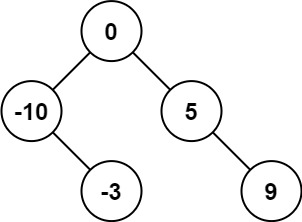Coding

# LeetCode Convert Sorted Array to Binary Search Tree Solution

0
(0)

Given an integer array `nums` where the elements are sorted in ascending order, convert it to a height-balanced binary search tree.

height-balanced binary tree is a binary tree in which the depth of the two subtrees of every node never differs by more than one.

Example 1:

```Input: nums = [-10,-3,0,5,9]
Output: [0,-3,9,-10,null,5]
Explanation: [0,-10,5,null,-3,null,9] is also accepted:```

Example 2:

```Input: nums = [1,3]
Output: [3,1]
Explanation: [1,null,3] and [3,1] are both height-balanced BSTs.
```

Constraints:

• `1 <= nums.length <= 104`
• `-104 <= nums[i] <= 104`
• `nums` is sorted in a strictly increasing order.

Solution

First let’s try to understand the problem statement. What the problem says that you are given an input of sorted array. Now you need to build a Binary Search Tree from elements of the array such that the result BST (Binary Search Tree) is height balanced. What is height balanced tree. A height balanced tree is such that at any node, difference between height of right sub trees and left trees is never more than 1.

Once I understood the problem statement, I started of thinking solutions approach for it. I thought for some time and then took out my biggest coding weapon for solving Binary Tree problems, yes you guessed it right, it was recursion. 🙂

The solution I thought can be described as follows. Choose the middle element of the array. Since the array is sorted, so we can safely assume that this middle element can become root of our balanced BST. Then divide the array into two arrays, first array would be having elements from 0 to index middle-1 and second array would be having elements from middle+1 to end of original array. Now choose middle element of first array. It will be left child of our root element. Similarly choose middle element of second array. It will be right child of our root element. Again do the same dividing of left and right sub arrays into two arrays each around their middle elements and so on. At the end you will get you balanced binary search tree. Think why you are getting a balanced binary tree, because you are always choosing mid element as root of the sorted array input.

Below is the java code for the same.

`````` public TreeNode sortedArrayToBST(int[] nums) {
int len = nums.length;
int mid = len/2;
TreeNode root = new TreeNode(nums[mid]);
TreeNode leftSubTree = sortedArrToBST(nums, 0, mid-1);
TreeNode rightSubTree = sortedArrToBST(nums, mid+1, len-1);
root.left = leftSubTree;
root.right = rightSubTree;
return root;
}

public TreeNode sortedArrToBST(int[] nums, int beg, int end) {
if (beg>end || beg>=nums.length) return null;
else if (beg == end) return new TreeNode(nums[beg]);
int mid = (beg + end)/2;
TreeNode root = new TreeNode(nums[mid]);
root.left = sortedArrToBST(nums, beg, mid-1);
root.right = sortedArrToBST(nums, mid+1, end);
return root;
}``````

And below is the efficiency. So it is faster than any solution submitted on LeetCode. 🙂

Lessons Learnt

• Recursion is a trick that is almost always useful in solving Binary Search Tree Problems.
• Whenever you see slightly tricky problems like this, your mind will give you two signals. One signal would be that you won’t be able to solve it, so just give up and look for solutions on the internet. The other signal would be that you can solve it and your mind will give you slight ideas/hint. Always follow the second signal and you will be able to solve the problem.

Click on a star to rate it!

Average rating 0 / 5. Vote count: 0

No votes so far! Be the first to rate this post.

As you found this post useful...

We are sorry that this post was not useful for you!

Let us improve this post!

Tell us how we can improve this post?